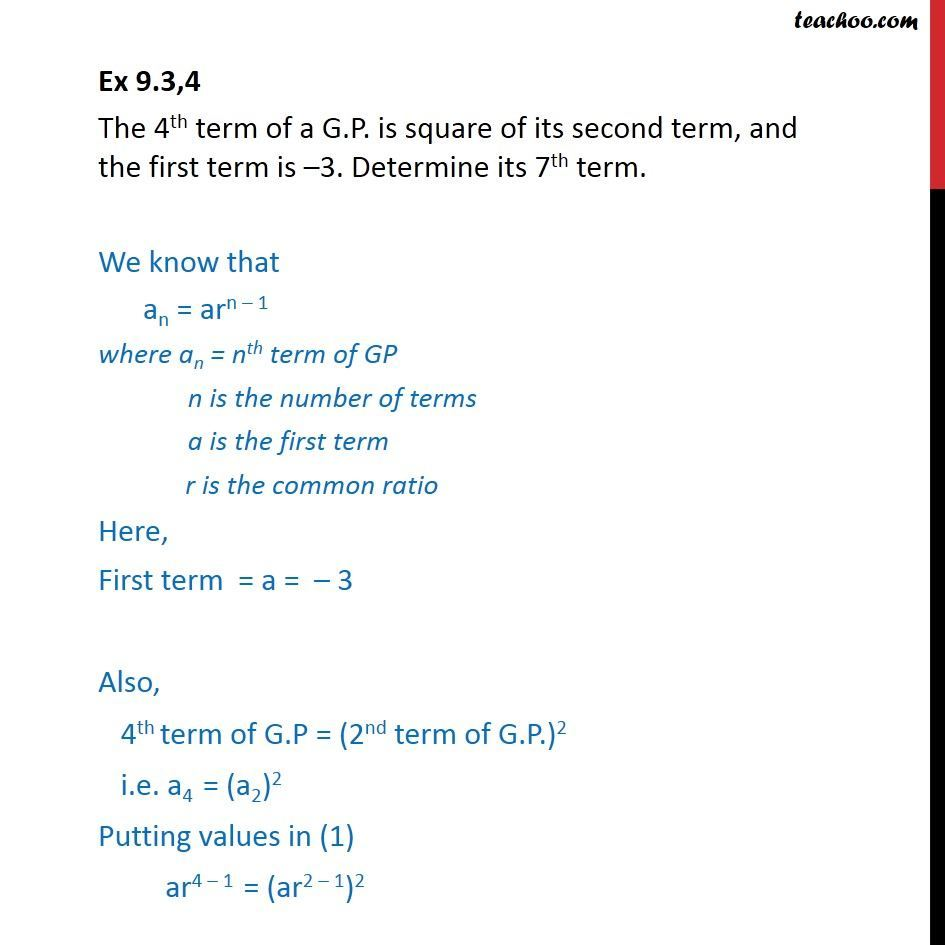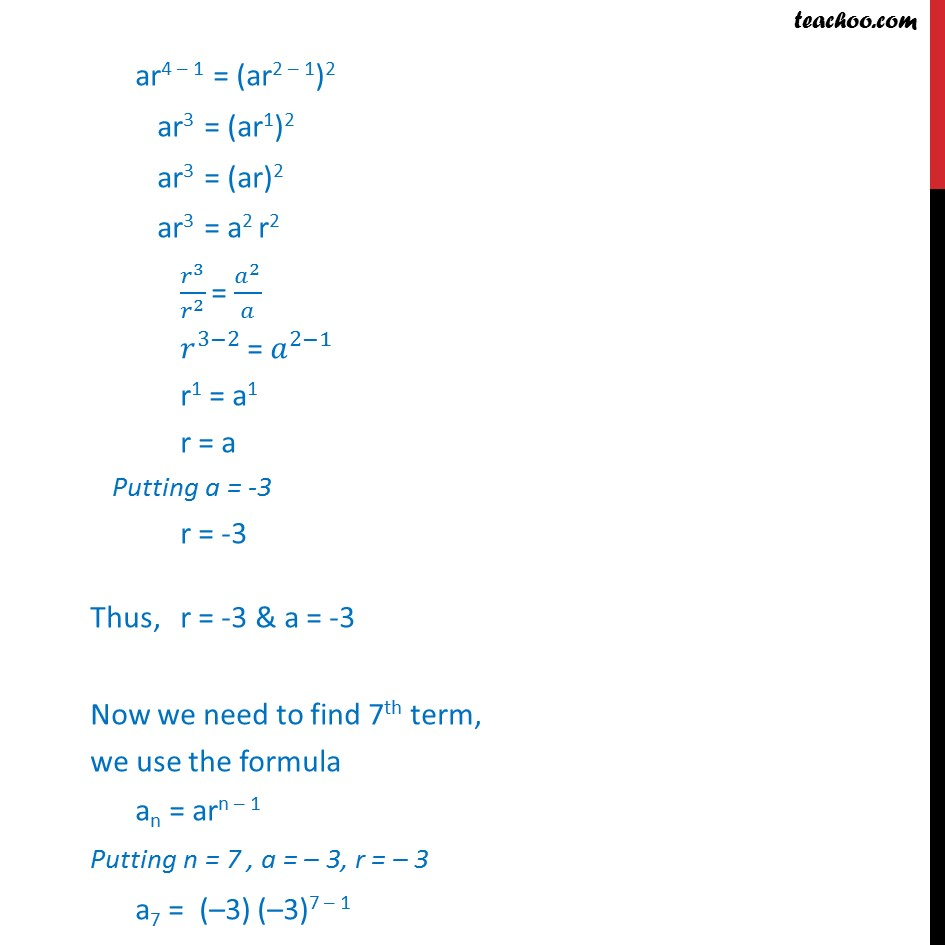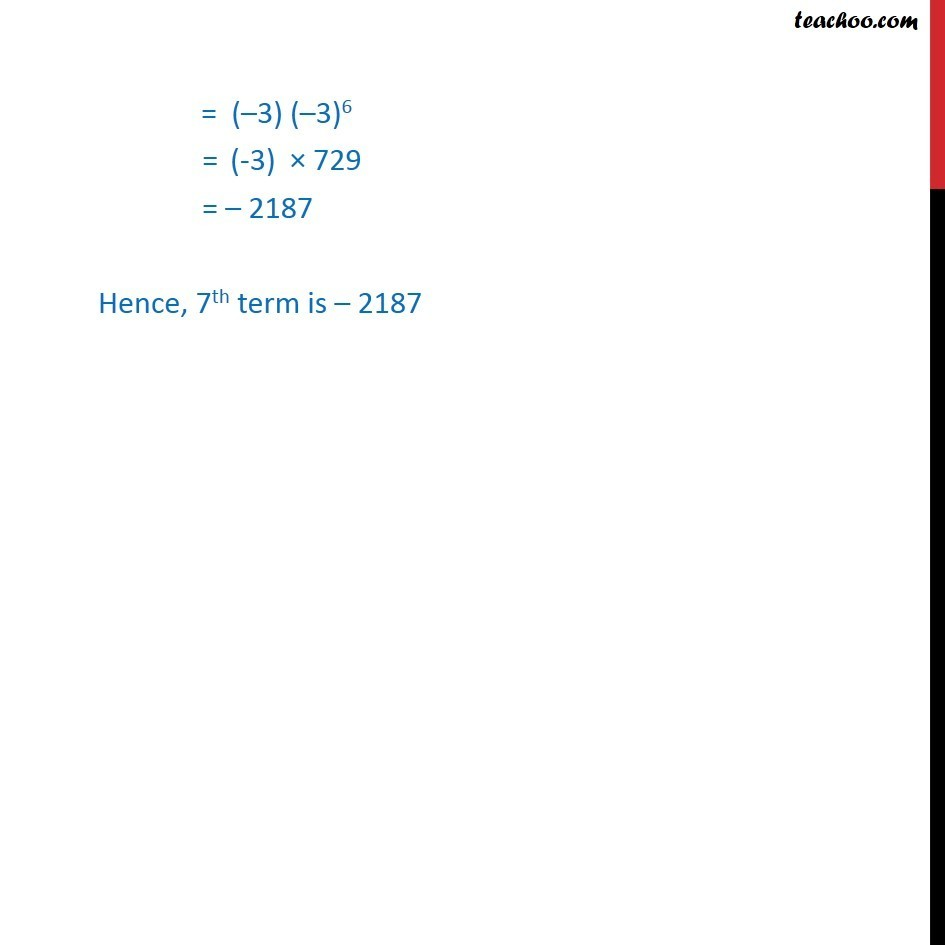Geometric Progression(GP): Formulae based

Chapter 8 Class 11 Sequences and Series
Concept wiseLearn in your speed, with individual attention - Teachoo Maths 1-on-1 Class

### Transcript

Ex 8.2,4 The 4th term of a G.P. is square of its second term, and the first term is 3. Determine its 7th term. We know that an = arn 1 where an = nth term of GP n is the number of terms a is the first term r is the common ratio Here, First term = a = 3 Also, 4th term of G.P = (2nd term of G.P.)2 i.e. a4 = (a2)2 Putting values in (1) ar4 1 = (ar2 1)2 ar3 = (ar1)2 ar3 = (ar)2 ar3 = a2 r2 ^3/ ^2 = ^2/ ^(3 2) = ^(2 1) r1 = a1 r = a Putting a = -3 r = -3 Thus, r = -3 & a = -3 Now we need to find 7th term, we use the formula an = arn 1 Putting n = 7 , a = 3, r = 3 a7 = ( 3) ( 3)7 1 = ( 3) ( 3)6 = (-3) 729 = 2187 Hence, 7th term is 2187